Work, Energy, and Power - Lesson 2 - The Work-Energy Relationship

# Analysis of Situations Involving External Forces

The previous part of Lesson 2 discussed the relationship between work and energy change. Whenever work is done upon an object by an external force, there will be a change in the total mechanical energy of the object. If only internal forces are doing work (no work done by external forces), there is no change in total mechanical energy; the total mechanical energy is said to be conserved. Because external forces are capable of changing the total mechanical energy of an object, they are sometimes referred to as nonconservative forces. Because internal forces do not change the total mechanical energy of an object, they are sometimes referred to as conservative forces. In this part of Lesson 2, we will further explore the quantitative relationship between work and energy.

### Relating Work to Energy

The quantitative relationship between work and mechanical energy is expressed by the following equation:

TMEi + Wext = TMEf

The equation states that the initial amount of total mechanical energy (TMEi) plus the work done by external forces (Wext) is equal to the final amount of total mechanical energy (TMEf). A few notes should be made about the above equation. First, the mechanical energy can be either potential energy (in which case it could be due to springs or gravity) or kinetic energy. Given this fact, the above equation can be rewritten as

KEi + PEi + Wext = KEf + PEf

The second note that should be made about the above equation is that the work done by external forces can be a positive or a negative work term. Whether the work term takes on a positive or a negative value is dependent upon the angle between the force and the motion. Recall from Lesson 1 that the work is dependent upon the angle between the force and the displacement vectors. If the angle is 180 degrees as it occasionally is, then the work term will be negative. If the angle is 0 degrees, then the work term will be positive.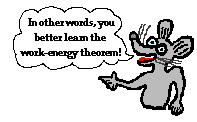The above equation is expresses the quantitative relationship between work and energy. This equation will be the basis for the rest of this unit. It will form the basis of the conceptual aspect of our study of work and energy as well as the guiding force for our approach to solving mathematical problems. A large slice of the world of motion can be understood through the use of this relationship between work and energy.

### Raising a Barbell Vertically

To begin our investigation of the work-energy relationship, we will investigate situations involving work being done by external forces (nonconservative forces). Consider a weightlifter who applies an upwards force (say 1000 N) to a barbell to displace it upwards a given distance (say 0.25 meters) at a constant speed. The initial energy plus the work done by the external force equals the final energy. If the barbell begins with 1500 Joules of energy (this is just a made up value) and the weightlifter does 250 Joules of work (F•d•cosine of angle = 1000 N•0.25 m•cosine 0 degrees = 250 J), then the barbell will finish with 1750 Joules of mechanical energy. The final amount of mechanical energy (1750 J) is equal to the initial amount of mechanical energy (1500 J) plus the work done by external forces (250 J).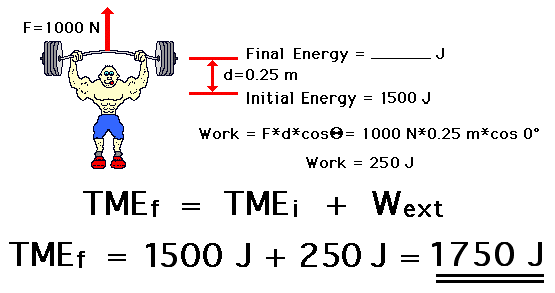### Catching a Baseball

Now consider a baseball catcher who applies a rightward force (say 6000 N) to a leftward moving baseball to bring it from a high speed to a rest position over a given distance (say 0.10 meters). The initial energy plus the work done by the external force equals the final energy. If the ball begins with 605 Joules of energy (this is just another made up value), and the catcher does -600 Joules of work (F•d•cosine of angle = 6000 N•0.10 m•cosine 180 degrees = -600 J), then the ball will finish with 5 Joules of mechanical energy. The final energy (5 J) is equal to the initial energy (605 J) plus the work done by external forces (-600 J).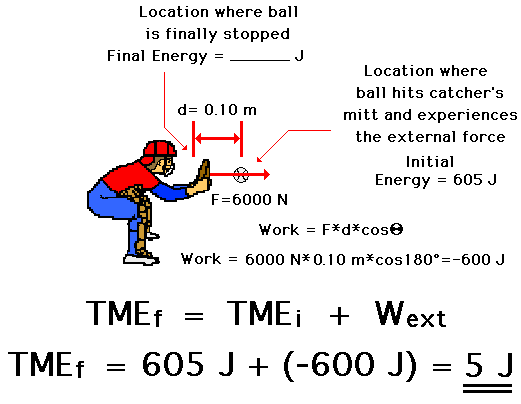### A Skidding Car

Now consider a car that is skidding from a high speed to a lower speed. The force of friction between the tires and the road exerts a leftward force (say 8000 N) on the rightward moving car over a given distance (say 30 m). The initial energy plus the work done by the external force equals the final energy. If the car begins with 320 000 Joules of energy (this is just another made up value), and the friction force does -240 000 Joules of work (F•d•cosine of angle = 8000 N•30 m•cosine 180 degrees = -240 000 J), then the car will finish with 80 000 Joules of mechanical energy. The final energy (80 000 J) is equal to the initial energy (320 000 J) plus the work done by external forces (-240 000 J).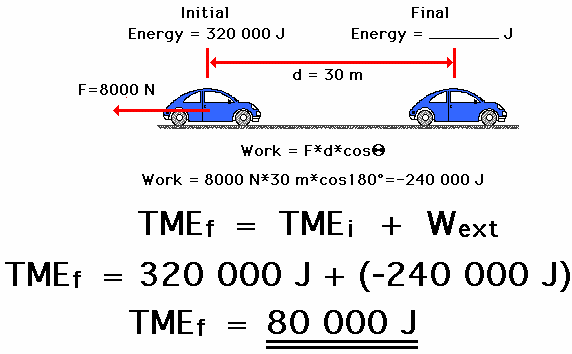### Pulling a Cart Up an Incline at Constant Speed

As a final example, consider a cart being pulled up an inclined plane at constant speed by a student during a Physics lab. The applied force on the cart (say 18 N) is directed parallel to the incline to cause the cart to be displaced parallel to the incline for a given displacement (say 0.7 m). The initial energy plus the work done by the external force equals the final energy. If the cart begins with 0 Joules of energy (this is just another made up value), and the student does 12.6 Joules of work (F•d•cosine of angle = 18 N•0.7 m•cosine 0 degrees = 12.6 J), then the cart will finish with 12.6 Joules of mechanical energy. The final energy (12.6 J) is equal to the initial energy (0 J) plus the work done by external forces (12.6 J).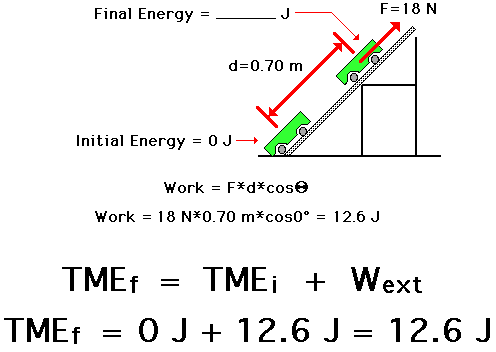In each of these examples, an external force does work upon an object over a given distance to change the total mechanical energy of the object. If the external force (or nonconservative force) does positive work, then the object gains mechanical energy. The amount of energy gained is equal to the work done on the object. If the external force (or nonconservative force) does negative work, then the object loses mechanical energy. The amount of mechanical energy lost is equal to the work done on the object. In general, the total mechanical energy of the object in the initial state (prior to the work being done) plus the work done equals the total mechanical energy in the final state.

###Your Turn to Try It

The work-energy relationship presented here can be combined with the expressions for potential and kinetic energy to solve complex problems. Like all complex problems, they can be made simple if first analyzed from a conceptual viewpoint and broken down into parts. In other words, avoid treating work-energy problems as mere mathematical problems. Rather, engage your mind and utilize your understanding of physics concepts to approach the problem. Ask "What forms of energy are present initially and finally?" and "Based on the equations, how much of each form of energy is present initially and finally?" and "Is work being done by external forces?" Use this approach on the following three practice problems. After solving, click the button to view the answers.

### We Would Like to Suggest ...Sometimes it isn't enough to just read about it. You have to interact with it! And that's exactly what you do when you use one of The Physics Classroom's Interactives. We would like to suggest that you combine the reading of this page with the use of our It's All Uphill Interactive and/or our Stopping Distance Interactive. Both Interactives can be found in the Physics Interactive section of our website and provide an interactive opportunity to explore the meaning of the work-energy relationship.

Practice Problem #1A 1000-kg car traveling with a speed of 25 m/s skids to a stop. The car experiences an 8000 N force of friction. Determine the stopping distance of the car.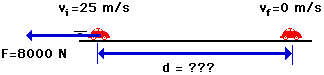Practice Problem #2At the end of the Shock Wave roller coaster ride, the 6000-kg train of cars (includes passengers) is slowed from a speed of 20 m/s to a speed of 5 m/s over a distance of 20 meters. Determine the braking force required to slow the train of cars by this amount.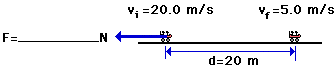Practice Problem #3A shopping cart full of groceries is sitting at the top of a 2.0-m hill. The cart begins to roll until it hits a stump at the bottom of the hill. Upon impact, a 0.25-kg can of peaches flies horizontally out of the shopping cart and hits a parked car with an average force of 500 N. How deep a dent is made in the car (i.e., over what distance does the 500 N force act upon the can of peaches before bringing it to a stop)?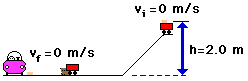### Stopping Distance

All three of the above problems have one thing in common: there is a force that does work over a distance in order to remove mechanical energy from an object. The force acts opposite the object's motion (angle between force and displacement is 180 degrees) and thus does negative work. Negative work results in a loss of the object's total amount of mechanical energy. In each situation, the work is related to the kinetic energy change. And since the distance (d) over which the force does work is related to the work and since the velocity squared (v^2) of the object is related to the kinetic energy, there must also be a direct relation between the stopping distance and the velocity squared. Observe the derivation below.

TMEi + Wext = TMEf

KEi + Wext = 0 J

0.5•m•vi2 + F•d•cos(Theta) = 0 J

0.5•m•vi2 = F•d

vi2d

The above equation depicts stopping distance as being dependent upon the square of the velocity. This means that a twofold increase in velocity would result in a fourfold (two squared) increase in stopping distance. A threefold increase in velocity would result in a nine-fold (three squared) increase in stopping distance. And a fourfold increase in velocity would result in a sixteen-fold (four squared) increase in stopping distance. This is one more example in which an equation becomes more than a mere algebraic recipe for solving problems. Equations can also be powerful guides to thinking about how two quantities are related to each other. In the case of a horizontal force bringing an object to a stop over some horizontal distance, the stopping distance of the object is related to the square of the velocity of the object.Test your understanding by predicting the stopping distance values in the table below (and see the practice problems at the end of this page).

 Velocity (m/s) Stopping Distance (m) 0 m/s 0 5 m/s 4 m 10 m/s See Answerd = 16 m From 5 m/s to 10 m/s is a two-fold increase (10 / 5 = 2) in velocity. Thus there should be a four-fold increase in stopping distance. Multiply 4 m by 4. 15 m/s See Answerd = 36 m From 5 m/s to 15 m/s is a three-fold increase (15 / 5 = 3) in velocity. Thus there should be a nine-fold increase in stopping distance. Multiply 4 m by 9. 20 m/s See Answerd = 64 m From 5 m/s to 20 m/s is a four-fold increase (20 / 5 = 4) in velocity. Thus there should be a 16-fold increase in stopping distance. Multiply 4 m by 16. 25 m/s See Answerd = 100 m From 5 m/s to 25 m/s is a five-fold increase (25 / 5 = 5) in velocity. Thus, there should be a 25-fold increase in stopping distance. Multiply 4 m by 25.

The above principle - that stopping distance is proportional to velocity squared - is often the focus of a popular physics lab. A Hot wheels car is rolled down an inclined plane to the floor below. Once reaching the floor, it strikes a computer diskette box and skids to a stop as a result of the friction between the car/box system and the floor. A photo gate time is used to determine the speed of the car prior to striking the box. Several trials are performed and a data set is collected and plotted. As the speed of the car is increased, the stopping distance is increased. If the data are plotted, then a clear power relationship is seen. If power regression is performed on the data set, the results tend to show that d = k•v2 where k is a constant of proportionality.The examples mentioned on this page involve the application of the work-energy relationship to situations involving external or nonconservative forces doing work. An entirely different outcome results in situations in which there is no work done by external forces. The next part of Lesson 2 involves an analysis of these situations.

Next Section: